Home » Polyhedra » Platonic Solids

# Volume of Platonic Solids

Platonic Solids, are a subcategory of the polyhedra. These geometric solids are the ones which each one of their faces are the same regular polygon, and at each vertex the same number of polygons are found.There are only 5 geometric solids considered Platonic solids.

Below you can select the three-dimensional geometric solid of your interest, inside you will find a description, the formula of the 3D geometric solid to calculate the volume, and you can also use a volume calculator to get the volume automatically.

## Geometric shapes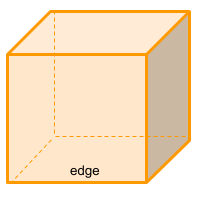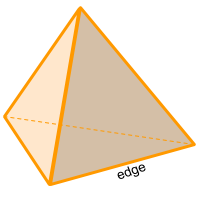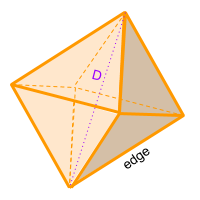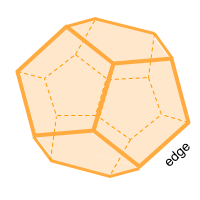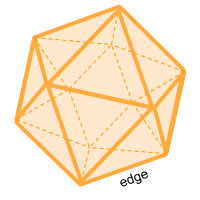Share